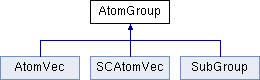ParM  parm A molecular dynamics library
AtomGroup Class Referenceabstract

a group of atoms, such as all of them (AtomVec), or a smaller group such as a molecule, sidebranch, etc. More...

#include <box.hpp>

Inheritance diagram for AtomGroup:## Public Member Functions

virtual AtomVecvec ()=0

virtual Atomoperator[] (cuint n)=0

virtual Atomoperator[] (cuint n) const =0

virtual Atomget (cuint n)

virtual AtomID get_id (cuint n)=0

virtual uint size () const =0
Number of atoms in the group. More...

virtual AtomIter begin ()
For use in a for loop. More...

virtual AtomIter end ()

Vec com () const
center of mass More...

Vec com_force () const
center of mass force (i.e., sum of all forces) More...

Vec com_velocity () const
center of mass velocity More...

flt mass () const
Mass of the whole group. More...

flt kinetic_energy (const Vec originvelocity=Vec::Zero()) const
Total kinetic_energy energy of the group. More...

Vec momentum () const
Total momentum. More...

$$R_g$$ More...

Vec torque (const Vec loc) const
Total torque about a given location. More...

Vec torque () const

flt moment_about (const Vec axis, const Vec loc) const
Moment of inertia of the atoms about an axis. More...

flt moment_about (const Vec axis) const

Vec angular_momentum (const Vec loc) const
Angular momentum. More...

Vec angular_momentum () const

Matrix moment (const Vec loc) const
Moment of inertia of the atoms as a whole. More...

Matrix moment () const

Vec omega (const Vec loc) const
Angular velocity. More...

Vec omega () const

void add_omega (Vec w, Vec origin)
Add a given angular velocity to all atoms, by adding to their velocity. More...

void reset_L ()
Reset angular momentum to 0. More...

for resetting. Adds a fixed velocity to all atoms More...

void reset_com_velocity ()
Subtracts the center of mass velocity from all atoms. More...

void randomize_velocities (flt T)
Randomize velocities, for a specific temperature. More...

void reset_forces ()
for timestepping More...

virtual ~AtomGroup ()

## Detailed Description

a group of atoms, such as all of them (AtomVec), or a smaller group such as a molecule, sidebranch, etc.

## Constructor & Destructor Documentation

 virtual AtomGroup::~AtomGroup ( )
inlinevirtual

## Member Function Documentation

 void AtomGroup::add_omega ( Vec w, Vec origin )

Add a given angular velocity to all atoms, by adding to their velocity.

 void AtomGroup::add_omega ( Vec w )
inline
 void AtomGroup::add_velocity ( Vec v )

for resetting. Adds a fixed velocity to all atoms

 Vec AtomGroup::angular_momentum ( const Vec loc ) const

Angular momentum.

 Vec AtomGroup::angular_momentum ( ) const
inline
 virtual AtomIter AtomGroup::begin ( )
inlinevirtual

For use in a for loop.

 Vec AtomGroup::com ( ) const

center of mass

 Vec AtomGroup::com_force ( ) const

center of mass force (i.e., sum of all forces)

 Vec AtomGroup::com_velocity ( ) const

center of mass velocity

 virtual AtomIter AtomGroup::end ( )
inlinevirtual
 virtual Atom& AtomGroup::get ( cuint n )
inlinevirtual

Reimplemented in SubGroup.

 virtual AtomID AtomGroup::get_id ( cuint n )
pure virtual

Implemented in SCAtomVec, SubGroup, and AtomVec.

$$R_g$$

 flt AtomGroup::kinetic_energy ( const Vec originvelocity = Vec::Zero() ) const

Total kinetic_energy energy of the group.

This is normally with reference to a "lab" reference frame (velocity (0,0,0)), but can optionally take a different origin velocity, e.g. com_velocity().

 flt AtomGroup::mass ( ) const

Mass of the whole group.

 Matrix AtomGroup::moment ( const Vec loc ) const

Moment of inertia of the atoms as a whole.

 Matrix AtomGroup::moment ( ) const
inline
 flt AtomGroup::moment_about ( const Vec axis, const Vec loc ) const

Moment of inertia of the atoms about an axis.

 flt AtomGroup::moment_about ( const Vec axis ) const
inline
 Vec AtomGroup::momentum ( ) const

Total momentum.

 Vec AtomGroup::omega ( const Vec loc ) const

Angular velocity.

 Vec AtomGroup::omega ( ) const
inline
 virtual Atom& AtomGroup::operator[] ( cuint n )
pure virtual

Implemented in SCAtomVec, SubGroup, and AtomVec.

 virtual Atom& AtomGroup::operator[] ( cuint n ) const
pure virtual

Implemented in SCAtomVec, SubGroup, and AtomVec.

 void AtomGroup::randomize_velocities ( flt T )

Randomize velocities, for a specific temperature.

 void AtomGroup::reset_com_velocity ( )
inline

Subtracts the center of mass velocity from all atoms.

 void AtomGroup::reset_forces ( )

for timestepping

 void AtomGroup::reset_L ( )
inline

Reset angular momentum to 0.

 virtual uint AtomGroup::size ( ) const
pure virtual

Number of atoms in the group.

Implemented in SCAtomVec, SubGroup, and AtomVec.

 Vec AtomGroup::torque ( const Vec loc ) const

Total torque about a given location.

 Vec AtomGroup::torque ( ) const
inline
 virtual AtomVec& AtomGroup::vec ( )
pure virtual

Implemented in SCAtomVec, SubGroup, and AtomVec.

The documentation for this class was generated from the following files: